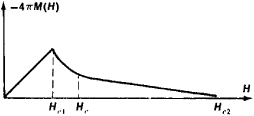# Which part of a real Type II Superconductor magnetization loop represents the Meissner state?

So if we consider an ideal type II superconductor the magnetization should look like thisThe Meissner state is to be found between $$0$$ and $$H_{c1}$$ and the superconductor works as an ideal diamagnet in this region. Between $$H_{c1}$$ and $$H_{c2}$$ is the mixed state where the vortices dominate the superconducting behaviour.

Now if we look at magnetization loops of real Type II superconductors then we see something like this(source: scielo.br)

[Braz. J. Phys. vol.32 no.3 São Paulo Sept. 2002]

After the first loop we dont see here any ideal diamagnetic curve anymore (like the one represented as a straight line in the first picture).

But is the Meissner state still located in the region between $$0$$ and $$H_{c1}$$ or is it even existing in real Type II superconductors (how they are found in reality)?